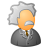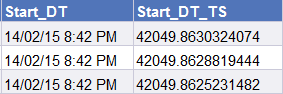# QlikView App Dev

Discussion Board for collaboration related to QlikView App Development.

cancel
Showing results for
Did you mean:Contributor III

## Excel Load Compare Dates in WHERE

Hello,

I see this question earlier,  Compare dates in WHERE clause while loading data from EXCEL however I am experiencing a similar problem whereby I cannot successfully compare dates in my Where clause. The intent is to load only records beyond a given date. In order to test that logic I am loading the last date and then all records with that date. There should only be one...however it returns 0.

MaxDT:
FROM
[myfile.xlsx]
(
ooxml, embedded labels, table is Records)
;
Let Wh_DateN = Peek('A');
Let Wh_DateS = date(Wh_DateN,'DD/MM/YYYY hh:mm:ss');
Drop Table MaxDT;
Trace 'Date number is \$(Wh_DateN)';
Trace 'Date string is \$(Wh_DateS)';

XCS:
FROM
[myfile.xlsx]
(
ooxml, embedded labels, table is Records)
WHERE Start_DT = '\$(Wh_DateN)'
;

MaxDT << Records 1 lines fetched
'Date number is 42188.692824074'
'Date string is 03/07/2015 16:37:40'
XCS << Records 0 lines fetched

John

1 Solution

Accepted SolutionsContributor III
Author

Looks like this request is not as straight forward as it would appear. It appears that the problem may be with the default number format used by Qlik in evaluating the Excel timestamp. I implemented a workaround to force the source system (BOE WEBI) to provide the timestamp in numeric format.When pulling the new Start_DT_TS value with the existing LOAD statement I was still unable to find the same record in my second LOAD statement. Looking closer it appeared that Qlik was only pulling 9 decimal places. This resulted in an inaccurate timestamp value that could not be found in my second LOAD. SO I changed the LOAD to;

The resulting value could then be used (as is) in my WHERE statement successfully.

Let nNEWXC_STRT_TS = Peek('C');

FROM [myfile.xlsx] (ooxml, embedded labels, table is Records)
WHERE Start_DT_TS > '\$(nNEWXC_STRT_TS)'

I'll mark this as complete for those stumbling across similar conditions with Excel timestamps.

8 RepliesMVP

You want to compare with TimeStamp or Date? If Date, use as below

MaxDT:

FROM

[myfile.xlsx]

(ooxml, embedded labels, table is Records);

Let Wh_DateN = Peek('A');

Let Wh_DateS = Date(Wh_DateN,'DD/MM/YYYY');

Drop Table MaxDT;

Trace 'Date number is \$(Wh_DateN)';

Trace 'Date string is \$(Wh_DateS)';

XCS:

FROM

[myfile.xlsx]

(ooxml, embedded labels, table is Records)

WHERE Start_DT = '\$(Wh_DateN)';

If TimeStamp, use as below

MaxDT:

FROM

[myfile.xlsx]

(ooxml, embedded labels, table is Records);

Let Wh_DateN = Peek('A');

Let Wh_DateS = TimeStamp(Wh_DateN,'DD/MM/YYYY hh:mm:ss');

Drop Table MaxDT;

Trace 'Date number is \$(Wh_DateN)';

Trace 'Date string is \$(Wh_DateS)';

XCS:

FROM

[myfile.xlsx]

(ooxml, embedded labels, table is Records)

WHERE TimeStamp(Start_DT) = '\$(Wh_DateN)';Contributor III
Author

Thanks for the quick response. It is timestamp I am comparing. Using your suggestions these are my results

MaxDT_TS << Records 1 lines fetched
'Excel timestamp is 03/07/2015 4:37:40 PM'
'Formatted timestamp is 03/07/2015 16:37:40'
XCS << Records 0 lines fetched

The Where clause is using the timestamp (WHERE Timestamp(Start_DT) = '\$(Wh_DateN)' ) but is still returns 0 records. There has to be one, as it was loaded in MaxDT.

Any other suggestions?MVP

Change below

Let Wh_DateS = TimeStamp(Timestamp#(Wh_DateN,'DD/MM/YYYY hh:mm:ss TT'));Contributor III
Author

Here is a snapshot of the data using this load statement;

Start_DT, Timestamp(Start_DT) as Start_DT_TSUsing either in the subsequent where clause returns 0 results.MVP

Can you provide sample excel file please?Contributor III
Author

As requestedContributor III
Author

Looks like this request is not as straight forward as it would appear. It appears that the problem may be with the default number format used by Qlik in evaluating the Excel timestamp. I implemented a workaround to force the source system (BOE WEBI) to provide the timestamp in numeric format.When pulling the new Start_DT_TS value with the existing LOAD statement I was still unable to find the same record in my second LOAD statement. Looking closer it appeared that Qlik was only pulling 9 decimal places. This resulted in an inaccurate timestamp value that could not be found in my second LOAD. SO I changed the LOAD to;

The resulting value could then be used (as is) in my WHERE statement successfully.

Let nNEWXC_STRT_TS = Peek('C');